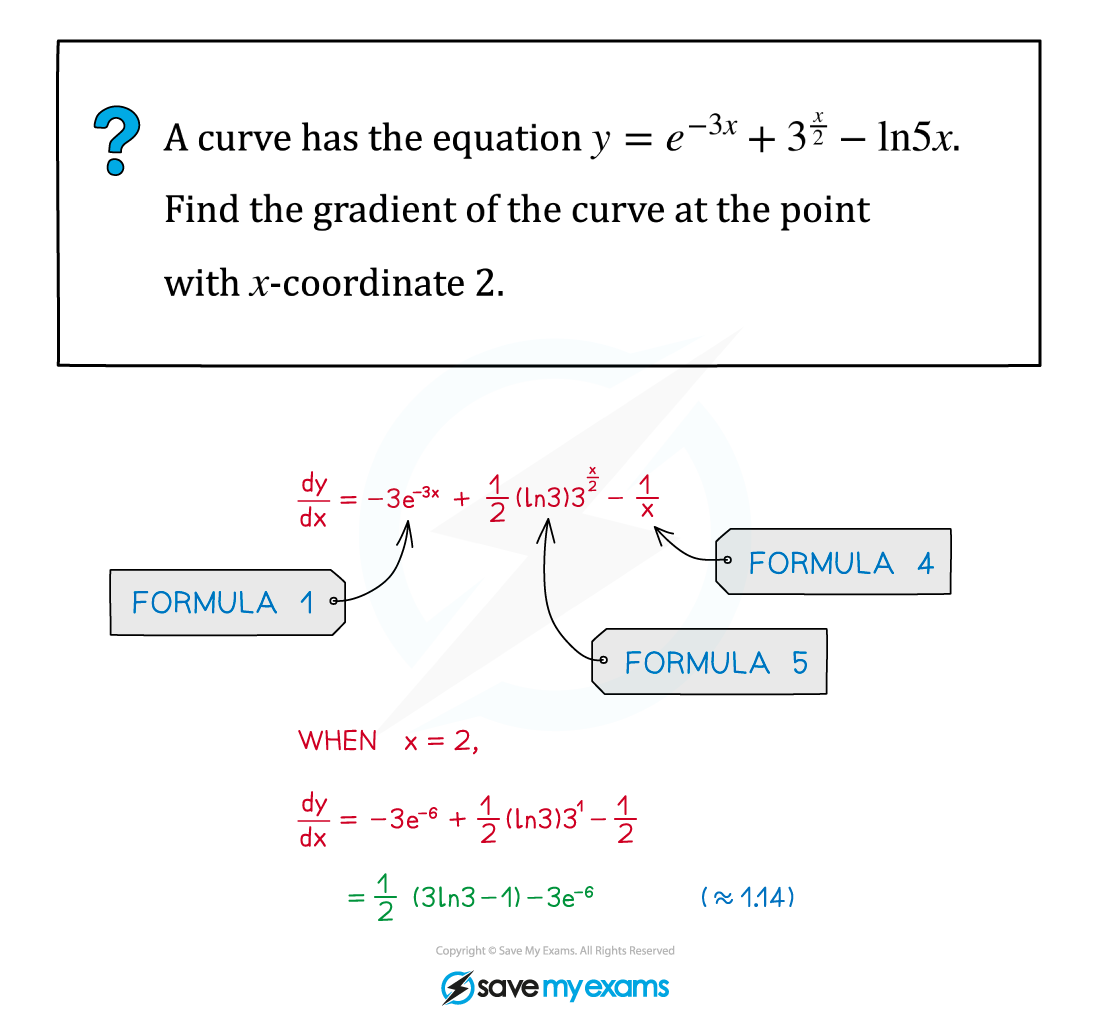# AQA A Level Maths: Pure复习笔记7.3.2 Differentiating Other Functions (Trig, ln & e etc)

### Differentiating Other Functions (Trig, ln & e etc)

#### How do I differentiate common functions?

• These are the common results

####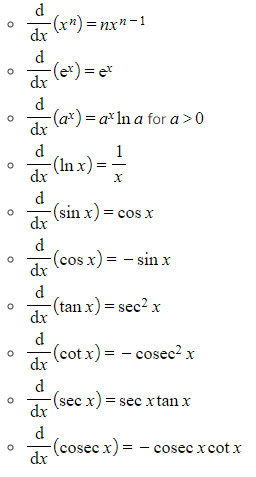How do I differentiate exponentials and logarithms?

• The two basic differentiation formulae are: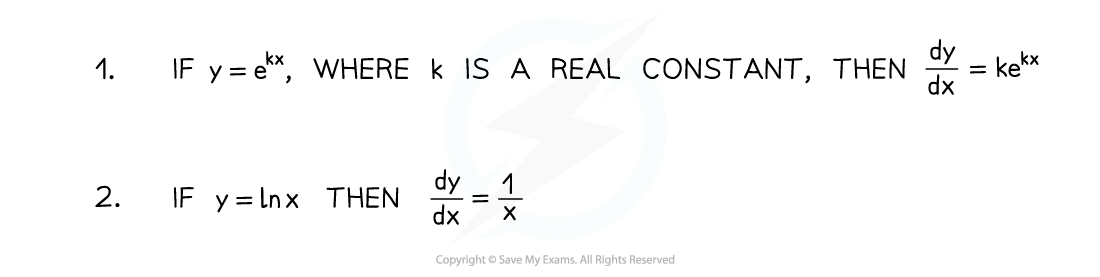• From those basic formulae are derived these two additional formulae: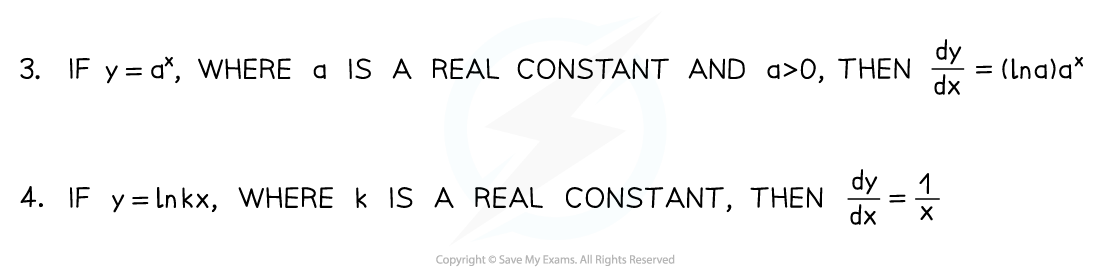• And for exponentials more generally:• This last formula can be derived from Formula 3 by using the chain rule

#### Exam Tip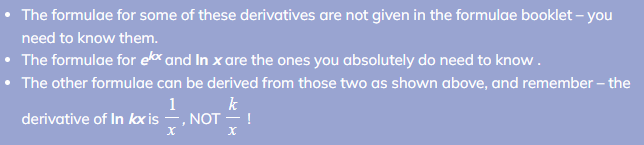Worked Example